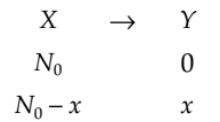Question

# A radioisotope X  with a half life 1.4 × ${10}^{9}$  years decays to Y  which is stable. A sample of the rock from a cave was found to contain X and Y in the ratio 1: 7 . The age of the rock is

Easy
Solution

## Number of Nuclei at t = 0Number of Nuclei at time tAs per question$\frac{{N}_{0}-x}{x}=\frac{1}{7}$$={N}_{0}-x={N}_{0}-\frac{7}{8}{N}_{0}=\frac{1}{8}{N}_{0}={\left(\frac{1}{2}\right)}^{3}{N}_{0}$So three half lives would have been passed

Get Instant Solutions